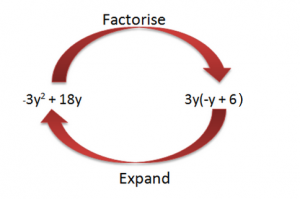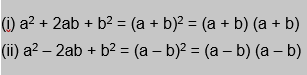Learn US

# How to factorize a polynomial? Part-2

155 views

1 October  2020

## Introduction

This blog is a sequel to the Basics of Factorization of Polynomials. The basics talk about polynomials and the first 2 methods of factorization, while this blog will talk about 3 other factoring methods.

• Factoring by applying Grouping Technique
• Factoring by Perfect Square Trinomial Method
• Factoring by Difference of Squares Method## Factoring by applying Grouping Technique

Regrouping refers to the rearrangement of the polynomial, by finding common terms.

 Example 1

a2 + ab + 8b
= a (a + b) + 8(a + b)
= (a + b) (a + 8)

 Example 2

15ab - 6a + 5b -2
= 3a (5b - 2) + 1 (5b -2)
= (3a + 1) (5b - 2)

Example question on Factoring Quadratic equations

ax2 + bx + c = 0
4x2 - 4x - 15 = 0

Find the factors of ac that add to b .

ac = 4(-15) = -60
b = -10 + 6 = -4

Write bx as the sum or difference using factors from step 1.
-4x = -10x + 6x

Divide the polynomials into two groups.
4x2 - 10x + 6x -15

Factor out GCF of the 1st half and factor out GCF of the 2nd half.

2x (2x - 5) + 3 (2x - 5)

Factor out the common binomial factor.
(2x - 5) (2x - 3)

## Factoring by Perfect Square Trinomial Method

To factorize a perfect square trinomial, we need to follow the steps given below:

• Identification of the squared numbers in the first and the third terms of the trinomial.
• Examination of the middle term to check whether it is positive or negative. Accordingly, the factors will have a plus and minus sign respectively.
• Writing the terms by applying the following identities:• So, an expression obtained from the square of the binomial equation is a perfect square trinomial.

An expression is called A PERFECT SQUARE TRINOMIAL if it takes the form ax2 + bx + c and satisfies the condition b2 = 4ac

Hence, the PERFECT SQUARE FORMULA takes the following forms:

(ax)2 + 2abx + b2 = (ax + b)2
(ax)2 −2abx + b2 = (ax − b)2

 Example

Factorize x2+ 6x + 9

### Solution:

We can rewrite the expression x2 + 6x + 9 in the form a2 + 2ab + b2 as;

x2+ 6x + 9 ⟹ (x)2 + 2 (x) (3) + (3)2

Applying the formula of a2 + 2ab + b2 = (a + b)2 to the expression gives;

= (x + 3)2
= (x + 3) (x + 3)

## Factoring by Difference of Squares Method

To factorize by the difference of squares, we need to follow the steps given below:

• Check if the four terms have anything in common (GCF). If yes, factor out the GCF. This GCF would be included in the final answer.
• Every difference of squares problem can be factored as follows:

a2 – b2 = (a + b) (a – b) or (a – b) (a + b).
So, we have to see what numbers squares will produce the desired results.

• Check whether the remaining factors can be factored any further or not.
 Example

x2 - 36

Step 1: Notice whether the four terms have anything in common, the GCF. If yes, factor the GCF out. Remember the GCF while noting down the final answer. In this case, the GCF is 1, which is of no use.

= x2 - 36

Step 2: Determine which term forms x2 and which number squared equals 36 to express in the form of (a + b)(a - b). In this case it is (x)(x) = x2 and (6)(6) = 36.

= x2 - 62

Step 3: Check for any possibility of further factorization. In this polynomial, there is no such opportunity.

= (x - 6) (x + 6)

## Summary

This blog covers in-depth 3 methods of Factoring Polynomials with details on each of them. It is a sequel to Factoring Polynomials - 1, which talks about the basics of polynomials and two methods to factorize them. This blog helps understand a stepwise breakdown of each method.

Cuemath, a student-friendly mathematics and coding platform, conducts regular Online Live Classes for academics and skill-development, and their Mental Math App, on both iOS and Android, is a one-stop solution for kids to develop multiple skills. Understand the Cuemath Fee structure and sign up for a free trial.

## What is Factoring by Grouping?

Regrouping refers to the rearrangement of the polynomial, by finding common terms. Therefore to factor a polynomial by grouping we group similar terms together, as shown above with examples.

## What is GCF?

GCF or the Greatest Common Factor is the largest quantity or term that is a factor of all the integers or polynomials involved.

The steps involved in finding the GCF of a given list of integers or polynomial terms are:

1. Prime factorize all the numbers
2. Identify the common prime factors
3. Take the product of all common prime factors
4. If there are no common prime factors, then the GCF is 1

## What are the different methods of factoring?

There are 5 different factoring methods:

1. Factorizing a Polynomial by Factoring out the HCF or GCF
2. Factorizing a Polynomial by Factor Theorem
3. Factoring by applying Grouping Technique
4. Factoring by Perfect Square Trinomial Method
5. Factoring by Difference of Squares Method

Written by Gurpreet Khatter, Cuemath Teacher

## External References

GIVE YOUR CHILD THE CUEMATH EDGE
Access Personalised Math learning through interactive worksheets, gamified concepts and grade-wise courses